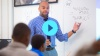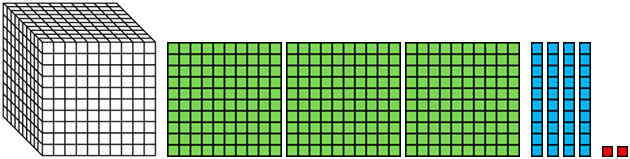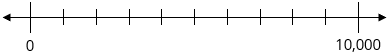# Place Value with Decimals

Students build upon their understanding of the place value system by extending its patterns to decimals, and continue to read, write, compare, and round numbers, including decimals, in various forms.

Math

Unit 1

## Unit Summary

In the first unit of 5th grade, students will build on their understanding of the structure of the place value system from 4th grade (MP.7) by extending that understanding to decimals. By the end of the unit, students will have a deep understanding of the base-ten structure of our number system, as well as how to read, write, compare, and round those numbers.

In 4th Grade Math, students developed the understanding that a digit in any place represents ten times as much as it represents in the place to its right (4.NBT.1). With this deepened understanding of the place value system, students read and wrote multi-digit whole numbers in various forms, compared them, and rounded them (4.NBT.2—3).

Unit 1 starts off with reinforcing some of this place value understanding of multi-digit whole numbers to 1 million, building up to that number by multiplying 10 by itself repeatedly. After this repeated multiplication, students are introduced to exponents to denote powers of 10. Then, students review the relationship in a whole number between a place value and the place to its left (4.NBT.1) and learn about the reciprocal relationship of a place value and the place to its right (5.NBT.1). Students also extend their work from 4th Grade Math on multiplying whole numbers by 10 to multiplying and dividing them by powers of 10 (5.NBT.2). After extensive practice with whole numbers, students then divide by 10 repeatedly to extend their place value system in the other direction, to decimals. They then apply these rules and perform these operations with powers of 10 to decimal numbers. Lastly, after deepening their understanding of the base-ten structure of our place value system, students read, write, compare, and round numbers in various forms (5.NBT.3—4).

As mentioned earlier, students will look for and make use of structure throughout the unit (MP.7). Students will also have an opportunity to look for and express regularity in repeated reasoning (MP.8), such as “when students explain patterns in the number of zeros of the product when multiplying a number by powers of 10 (5.NBT.2)” (PARCC Model Content Frameworks, p. 24).

This content represents the culmination of many years’ worth of work to deeply understand the structure of our place value system, starting all the way back in Kindergarten with the understanding of teen numbers as “10 ones and some ones” (K.NBT.1). Moving forward, students will rely on this knowledge later in 5th Grade Math to multiply and divide whole numbers (5.NBT.5—6) and perform all four operations with decimals (5.NBT.7). Students will also use their introduction to exponents to evaluate more complex expressions involving them (6.EE.1). Perhaps the most obvious future grade-level connection exists in 8th Grade Math, when students will represent very large and very small numbers using scientific notation and perform operations on numbers written in scientific notation (8.EE.3—4). Thus, this unit represents an important conclusion to the underlying structure of our number system and opens the door to more complex mathematics with very large and very small numbers.

Pacing: 16 instructional days (13 lessons, 2 flex days, 1 assessment day)

Fishtank Plus for Math

Unlock features to optimize your prep time, plan engaging lessons, and monitor student progress.## Assessment

The following assessments accompany Unit 1.

### Pre-Unit

Have students complete the Pre-Unit Assessment and Pre-Unit Student Self-Assessment before starting the unit. Use the Pre-Unit Assessment Analysis Guide to identify gaps in foundational understanding and map out a plan for learning acceleration throughout the unit.

### Mid-Unit

Have students complete the Mid-Unit Assessment after lesson 6.

### Post-Unit

Use the resources below to assess student understanding of the unit content and action plan for future units.

Expanded Assessment Package

Use student data to drive your planning with an expanded suite of unit assessments to help gauge students’ facility with foundational skills and concepts, as well as their progress with unit content.

## Unit Prep

### Intellectual Prep

Unit Launch

Prepare to teach this unit by immersing yourself in the standards, big ideas, and connections to prior and future content. Unit Launches include a series of short videos, targeted readings, and opportunities for action planning.#### Intellectual Prep for All Units

• Read and annotate “Unit Summary” and “Essential Understandings” portion of the unit plan.
• Do all the Target Tasks and annotate them with the “Unit Summary” and “Essential Understandings” in mind.
• Take the Post-Unit Assessment.

#### Unit-Specific Intellectual Prep

• Read the following table that includes models used in this unit.
Concrete or pictorial base ten blocksPlace value chart
 Ten thousands Thousands Hundreds Tens Ones 4 8 0 5 0
Number line### Essential Understandings

• A digit in any place represents 10 times as much as it represents in the place to its right and $${\frac{1}{10}}$$ of what it represents in the place to its left.
• Multiplying a number by 10 repeatedly (or a power of 10) results in the digits shifting to the left. The digits shift the same number of places as are factors of 10.
• Dividing a number by 10 repeatedly (or a power of 10) results in the digits shifting to the right. The digits shift the same number of places as are factors of 10.
• Comparing numbers written in standard form uses the understanding that one of any unit is greater than any amount of a smaller unit. Thus, the largest place values in each number contains the most relevant information when comparing numbers. If both numbers have the same number of largest units, the next largest place value should be attended to next, iteratively until one digit is greater than another in the same unit.
• When rounding a number, the goal is to approximate the number by the closest number with no units of smaller value (e.g., so 3.56 to the nearest tenth is 3.60; and to the nearest whole is 4). When a number that is being rounded has a 5 in the place being considered and 0 in all smaller places, it is equidistant from the two benchmarks. Therefore, it is simply a convention that the number is rounded to the greater benchmark.

### Materials

• Base ten blocks (Maximum of 8 thousands, 80 hundreds, 70 tens, and 80 ones per student or small group) — Students might not need this many depending on their reliance on concrete materials. You could just use one set for the teacher if materials are limited.
• Paper hundreds flats (20 count) (About 100 per class period)
• Tape or stapler (1 per small group)
• Millions Place Value Chart (Total of 10 per student) — Students might need more or less depending on their reliance on this tool.
• Thousands Place Value Chart (Total of 6 per student) — Students might need more or less depending on their reliance on this tool.

### Vocabulary

exponent

power

thousandth

To see all the vocabulary for Unit 1, view our 5th Grade Vocabulary Glossary.

## Unit Practice

Word Problems and Fluency Activities

Access daily word problem practice and our content-aligned fluency activities created to help students strengthen their application and fluency skills.## Lesson Map

Topic A: Place Value with Whole Numbers

Topic B: Place Value with Decimals

Topic C: Reading, Writing, Comparing, and Rounding Decimals

## Common Core Standards

Key

Major Cluster

Supporting Cluster

### Core Standards

#### Number and Operations in Base Ten

• 5.NBT.A.1 — Recognize that in a multi-digit number, a digit in one place represents 10 times as much as it represents in the place to its right and 1/10 of what it represents in the place to its left.
• 5.NBT.A.2 — Explain patterns in the number of zeros of the product when multiplying a number by powers of 10, and explain patterns in the placement of the decimal point when a decimal is multiplied or divided by a power of 10. Use whole-number exponents to denote powers of 10.
• 5.NBT.A.3 — Read, write, and compare decimals to thousandths.
• 5.NBT.A.3.A — Read and write decimals to thousandths using base-ten numerals, number names, and expanded form, e.g., 347.392 = 3 × 100 + 4 × 10 + 7 × 1 + 3 × (1/10) + 9 × (1/100) + 2 × (1/1000).
• 5.NBT.A.3.B — Compare two decimals to thousandths based on meanings of the digits in each place, using >, =, and < symbols to record the results of comparisons.
• 5.NBT.A.4 — Use place value understanding to round decimals to any place.

• 3.NBT.A.1
• 4.NBT.A.1
• 4.NBT.A.2
• 4.NBT.A.3

• 4.NF.B.4.A
• 4.NF.C.5
• 4.NF.C.6
• 4.NF.C.7

• 6.EE.A.1
• 8.EE.A.3

• 5.NBT.B.5
• 5.NBT.B.6
• 5.NBT.B.7

### Standards for Mathematical Practice

• CCSS.MATH.PRACTICE.MP1 — Make sense of problems and persevere in solving them.

• CCSS.MATH.PRACTICE.MP2 — Reason abstractly and quantitatively.

• CCSS.MATH.PRACTICE.MP3 — Construct viable arguments and critique the reasoning of others.

• CCSS.MATH.PRACTICE.MP4 — Model with mathematics.

• CCSS.MATH.PRACTICE.MP5 — Use appropriate tools strategically.

• CCSS.MATH.PRACTICE.MP6 — Attend to precision.

• CCSS.MATH.PRACTICE.MP7 — Look for and make use of structure.

• CCSS.MATH.PRACTICE.MP8 — Look for and express regularity in repeated reasoning.

Unit 2

Multiplication and Division of Whole Numbers

## Request a Demo

See all of the features of Fishtank in action and begin the conversation about adoption.

Yes

No

### Any other information you would like to provide about your school?